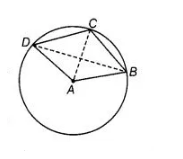# ABCD is such a quadrilateral that A is`
Question:

ABCD is such a quadrilateral that A is the centre of the circle passing through B, C and D. Prove that

Solution:

Given in a circle, $A B C D$ is a quadrilateral having centre $A$.

To prove  $\angle C B D+\angle C D B=\frac{1}{2} \angle B A D$

Construction Join $A C$ and $B D$.

Proof Since, arc $D C$ subtends $\angle D A C$ at the centre and $\angle C B D$ at a point $B$ in the remaining part of the circle.$\because$ $\angle D A C=2 \angle C B D \quad \ldots$ (i)

In a circle, the angle subtended by an arc at the centre is twice the angle subtended by it at the remaining part of the circle.

Similarly, arc $B C$ subtends $\angle C A B$ at the centre and $\angle C D B$ at a point $D$ in the remaining part of the circle.

$\therefore$ $\angle C A B=2 \angle C D B$ $\ldots$ (ii)

In a circle, the angle subtended by an arc at the centre is twice the angle subtended by it at the remaining part of the circle.

On adding Eqs. (i) and (ii), we get

$\angle D A C+\angle C A B=2 \angle C B D+2 \angle C D B$

$\Rightarrow \quad \angle B A D=2(\angle C B D+\angle C D B)$

$\Rightarrow \quad \angle C D B+\angle C B D=\frac{1}{2} \angle B A D$

Hence proved.